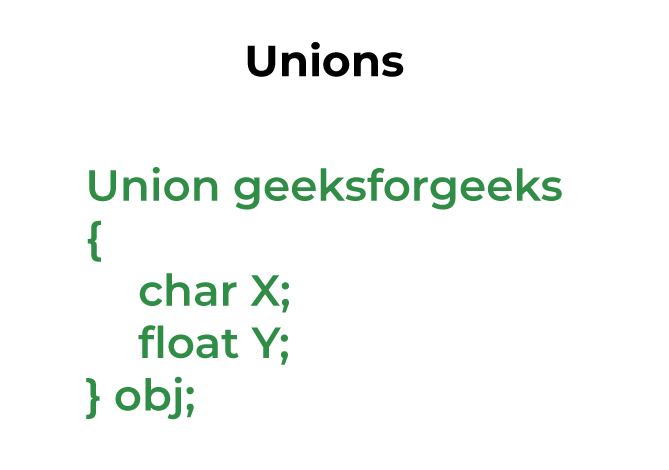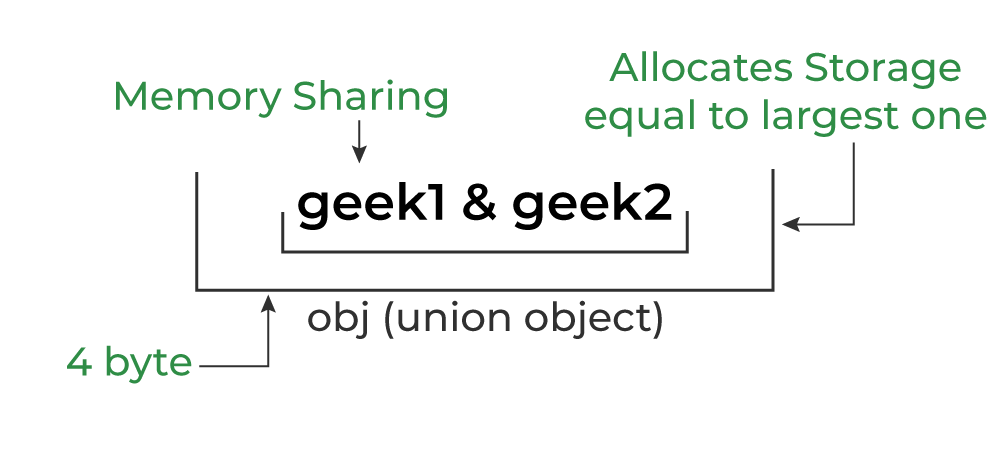GFG App
Open AppBrowser
Continue

# C Unions

The Union is a user-defined data type in C language that can contain elements of the different data types just like structure. But unlike structures, all the members in the C union are stored in the same memory location. Due to this, only one member can store data at the given instance.## Syntax of Union in C

The syntax of the union in C can be divided into three steps which are as follows:

### C Union Declaration

In this part, we only declare the template of the union, i.e., we only declare the members’ names and data types along with the name of the union. No memory is allocated to the union in the declaration.

```union union_name {
datatype member1;
datatype member2;
...
};```

Keep in mind that we have to always end the union declaration with a semi-colon.

### Different Ways to Define a Union Variable

We need to define a variable of the union type to start using union members. There are two methods using which we can define a union variable.

1. With Union Declaration
2. After Union Declaration

#### 1. Defining Union Variable with Declaration

```union union_name {
datatype member1;
datatype member2;
...
} var1, var2, ...;```

#### 2. Defining Union Variable after Declaration

`union union_name var1, var2, var3...;`

where union_name is the name of an already declared union.

### Access Union Members

We can access the members of a union by using the ( . ) dot operator just like structures.

`var1.member1;`

where var1 is the union variable and member1 is the member of the union.

The above method of accessing the members of the union also works for the nested unions.

`var1.member1.memberA;`

Here,

• var1 is a union member.
• member1 is a member of the union.
• memberA is a member of member1.

### Initialization of Union in C

The initialization of a union is the initialization of its members by simply assigning the value to it.

`var1.member1 = some_value;`

One important thing to note here is that only one member can contain some value at a given instance of time.

## C

 `// C Program to demonstrate how to use union ` `#include ` ` `  `// union template or declaration ` `union` `un { ` `    ``int` `member1; ` `    ``char` `member2; ` `    ``float` `member3; ` `}; ` ` `  `// driver code ` `int` `main() ` `{ ` ` `  `    ``// defining a union variable ` `    ``union` `un var1; ` ` `  `    ``// initializing the union member ` `    ``var1.member1 = 15; ` ` `  `    ``printf``(``"The value stored in member1 = %d"``, ` `           ``var1.member1); ` ` `  `    ``return` `0; ` `}`

Output

`The value stored in member1 = 15`

## Size of Union

The size of the union will always be equal to the size of the largest member of the array. All the less-sized elements can store the data in the same space without any overflow.Memory Allocation in C Union

## C

 `// C Program to find the size of the union ` `#include ` ` `  `// declaring multiple unions ` `union` `test1 { ` `    ``int` `x; ` `    ``int` `y; ` `} Test1; ` ` `  `union` `test2 { ` `    ``int` `x; ` `    ``char` `y; ` `} Test2; ` ` `  `union` `test3 { ` `    ``int` `arr; ` `    ``char` `y; ` `} Test3; ` ` `  `// driver code ` `int` `main() ` `{ ` `    ``// finding size using sizeof() operator ` `    ``int` `size1 = ``sizeof``(Test1); ` `    ``int` `size2 = ``sizeof``(Test2); ` `    ``int` `size3 = ``sizeof``(Test3); ` ` `  `    ``printf``(``"Sizeof test1: %d\n"``, size1); ` `    ``printf``(``"Sizeof test2: %d\n"``, size2); ` `    ``printf``(``"Sizeof test3: %d"``, size3); ` `    ``return` `0; ` `}`

Output

```Sizeof test1: 4
Sizeof test2: 4
Sizeof test3: 40```

## Difference between C Structure and C Union

The following table lists the key difference between the structure and union in C:

## FAQs on C Unions

### 1. What is the size of the given union?

```union un {
int a;
int arr;
}```

Ans: The size of the given union is 20 x 4 bytes = 80 bytes. Even if the array is a collection of similar data elements, it is considered to be a single entity by the C compiler.

### 2. Can we store data in multiple union members at the same time?

No. We can only store data in a single member at the same time. For example in the following C program, both x and y share the same location. If we change x, we can see the changes being reflected in y.

## C

 `// C program to check if we can store data in multiple union ` `// members ` `#include ` ` `  `// Declaration of union is same as structures ` `union` `test { ` `    ``int` `x, y; ` `}; ` ` `  `int` `main() ` `{ ` `    ``// A union variable t ` `    ``union` `test t; ` ` `  `    ``t.x = 2; ``// t.y also gets value 2 ` `    ``printf``(``"After making x = 2:\n x = %d, y = %d\n\n"``, t.x, ` `           ``t.y); ` ` `  `    ``t.y = 10; ``// t.x is also updated to 10 ` `    ``printf``(``"After making y = 10:\n x = %d, y = %d\n\n"``, t.x, ` `           ``t.y); ` `    ``return` `0; ` `}`

Output

```After making x = 2:
x = 2, y = 2

After making y = 10:
x = 10, y = 10```

### 3. What are the applications of unions?

Unions can be useful in many situations where we want to use the same memory for two or more members. For example, suppose we want to implement a binary tree data structure where each leaf node has a double data value, while each internal node has pointers to two children, but no data. If we declare this as:

## C

 `struct` `NODE { ` `    ``struct` `NODE* left; ` `    ``struct` `NODE* right; ` `    ``double` `data; ` `}; `

then every node requires 16 bytes, with half the bytes wasted for each type of node. On the other hand, if we declare a node as the following, then we can save space.

## C

 `struct` `NODE { ` `    ``bool` `is_leaf; ` `    ``union` `{ ` `        ``struct` `        ``{ ` `            ``struct` `NODE* left; ` `            ``struct` `NODE* right; ` `        ``} internal; ` `        ``double` `data; ` `    ``} info; ` `}; `

My Personal Notes arrow_drop_up